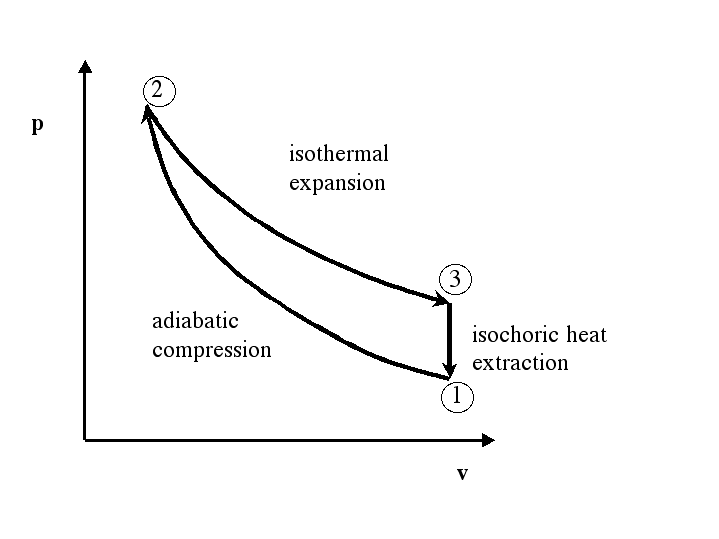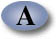UNIFIED ENGINEERING Fall 2004

Ian A. Waitz

Problem T6. (Thermodynamics)

Consider the following thermodynamic cycle. Assume all processes are quasi-static.Air undergoes a quasi-static thermodynamic cycle 1-2-3-1 as shown below. Process 1-2 is adiabatic compression, 2-3 is isothermal expansion, and process 3-1 is constant volume heat removal. The conditions at state 1 are p1 = 100kPa, T1=300K. The compression ratio of the cycle (v1/v2) is 8. Assume that cp = 1.0035 kJ/kg-K and cv = 0.7165 kJ/kg-K are constants, and that R = 0.287 kJ/kg-K.

a) For each leg of the cycle identify whether the heat added to the system, Q, and the work done by the system, W, are positive, negative or zero.

b) For each leg of the cycle calculate the work and heat transfer, the change in internal energy and the change in enthalpy.

c) What is the net work of the cycle?

d) What is the thermal efficiency of the cycle?